### Problem Set 1 Sets Class 9th Mathematics Part I MHB Solution

Problem Set 1 Sets Class 9th Mathematics Part I MHB Solution
###### Problem Set 1
Question 1.

Choose the correct alternative answer for each of the following questions.

If M = {1, 3, 5}, N = {2, 4, 6}, then M ∩ N =?
A. {1, 2, 3, 4, 5, 6}

B. {1, 3, 5}

C. ϕ

D. {2, 4, 6}

Given: M = {1, 3, 5}, N = {2, 4, 6}

(M ∩ N) = set of all common elements of M and N

Hence, (M ∩ N) = ϕ

Option (C) is correct.

Given : P = {x | x is an odd natural number,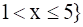set in roster form:

⇒ P = {3, 5}

Option (D) is correct.

Question 3.

Choose the correct alternative answer for each of the following questions.

P = {1, 2, ........., 10}, What type of set P is ?
A. Null set

B. Infinite set

C. Finite set

D. None of these

Given : P = {1, 2, ........., 10}

As the number of elements given are finite.

⇒ given set is finite set.

Option (C) is correct.

Question 4.

Choose the correct alternative answer for each of the following questions.

M ∪ N= {1, 2, 3, 4, 5, 6} and M = {1, 2, 4} then which of the following represent set N?
A. {1, 2, 3}

B. {3, 4, 5, 6}

C. {2, 5, 6}

D. {4, 5, 6}

Given : (M ∪ N) = {1, 2, 3, 4, 5, 6}, M = {1, 2, 4}

(M ∪ N) = set of all elements of set M and N

Hence, N = {3, 4, 5, 6}

Option (B) is correct.

Question 5.

Choose the correct alternative answer for each of the following questions.

If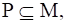then Which of the following set represent P ∩(P ∪ M)?
A. P

B. M

C. P ∪ M

D. P ∩ M

Given :As,Then using property,

(P ∪ M) = M

As,Then using property,

(P ∩ M) = P

Question 6.

Choose the correct alternative answer for each of the following questions.

Which of the following sets are empty sets?
A. set of intersecting points of parallel lines

B. set of even prime numbers.

C. Month of an english calendar having less than 30 days.

D.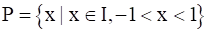A. set of intersecting points of parallel lines = ϕ

Because no two parallel lines will intersect.

Hence, Option (A) is correct.

Question 7.

Find the correct option for the given question.

Which of the following collections is a set?
A. Colours of the rainbow

B. Tall trees in the school campus.

C. Rich people in the village

D. Easy examples in the book

SET: If we can definitely and clearly decide the objects of a given collection

then that collection is called set.

Hence, option A is correct answer.

Because we can clearly say that colours of rainbow are seven in number as

(violet, indigo, blue, green, yellow, orange and red).

Hence, Option (A) is correct.

Question 8.

Find the correct option for the given question.

Which of the following set represent N ∩ W?
A. {1, 2, 3, .....}

B. {0, 1, 2, 3, ....}

C. {0}

(D) { }

Given : N = {1, 2, .........∞} and W = {0, 1, 2, .........∞}

(N ∩ W) = set of all common elements of N and W

Hence, (N ∩ W) = {1, 2, .........∞}

Option (A) is correct.

Question 9.

Find the correct option for the given question.

P = {x | x is a letter of the word ' Indian'} then which one of the following is set P in listing form?
A. {i, n, d}

B. {i, n, d, a}

C. {i,n,d,i,a}

D. {n, d, a}

Given : P = {x | x is a letter of the word ' indian'}

listing form = each of the element is written only once and separated by commas in curly brackets.

Hence, P = {i, n, d, a}

Option (B) is correct.

Question 10.

Find the correct option for the given question.

If T = {1, 2, 3, 4, 5} and M = {3, 4, 7, 8} then T ∪ M=?
A. {1, 2, 3, 4, 5, 7}

B. {1, 2, 3, 7, 8}

C. {1, 2, 3, 4, 5, 7, 8}

D. {3, 4}

Given : T = {1, 2, 3, 4, 5} and M = {3, 4, 7, 8}

(T ∪ M) = set of all elements of set T and M

Hence, (T ∪ M) = {1, 2, 3, 4, 5, 7, 8}

Option (C) is correct.

Question 11.

Out of 100 persons in a group, 72 persons speak English and 43 persons speak French. Each one out of 100 persons speak at least one language. Then how many speak only English? How many speak only French? How many of them speak English and French both?

Given: n(A) = 72, n(B) = 43 and n (A ∪ B) = 100

Total number of persons who speak at least one language = n (A ∪ B) = 100

n(A) = number of students who speaks English

n(B) = number of students who speaks French

n (A ∩ B) = number of students who speak both

As we know, n (A ∪ B) = n(A) + n(B) - n (A ∩ B)

⇒ n (A ∩ B) = 72 + 43 - 100

⇒ n (A ∩ B) = 115 - 100

⇒ n (A ∩ B) = 15

number of students who speak both the language = 15

number of students who speaks English only = 72 – 15 = 57

number of students who speaks French only = 43 – 15 = 28

Question 12.

70 trees were planted by Parth and 90 trees were planted by Pradnya on the occasion ofTree Plantation Week. Out of these; 25 trees were planted by both of them together. Howmany trees were planted by Parth or Pradnya?

Given: n(A) = 70, n(B) = 90 and n (A ∩ B) = x = 25

n(A) = number of trees planted by Parth

n(B) = number of trees planted by Pradnya

n (A ∩ B) = number of trees planted by both of them together

n (A ∪ B) = number of trees planted by Parth or Pradnya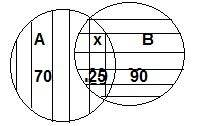As we know, n (A ∪ B) = n(A) + n(B) - n (A ∩ B)

⇒ n (A ∪ B) = 70 + 90 - 25

⇒ n (A ∪ B) = 160 - 25

⇒ n (A ∪ B) = 35

Question 13.

If n(A) = 20, n(B) = 28 and n (A ∪ B) = 36 then n (A ∩ B) = ?

Given: n(A) = 20, n(B) = 28 and n (A ∪ B) = 36

As we know, n (A ∪ B) = n(A) + n(B) - n (A ∩ B)

⇒ n (A ∩ B) = 20 + 28 - 36

⇒ n (A ∩ B) = 48 - 36

⇒ n (A ∩ B)) = 12

Question 14.

In a class, 8 students out of 28 have a dog as their pet animal at home, 6 students have a cat as their pet animal. 10 students have dog and cat both, then how many students do not have a dog or cat as their pet animal at home?

Given: n(A) = 8, n(B) = 6 and n (A ∩ B) = 10

n(A) = number of students having only dog as pet

n(B) = number of students having only cat as pet

n (A ∩ B) = number of trees planted by both of them together

Total students having a dog or cat as their pet animal at home = 8 + 6 + 10

= 24

Hence, number of students do not have a dog or cat as their pet animal at

Home = 28 – 24 = 4

Question 15.

Represent the union of two sets by Venn diagram for each of the following.

A = {3, 4, 5, 7}

B = {1, 4, 8}

Given: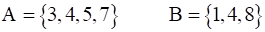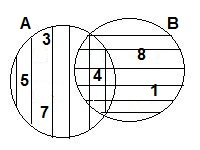Question 16.

Represent the union of two sets by Venn diagram for each of the following.

P = {a, b, c, e, f}

Q = {I, m, n, e, b}

Given: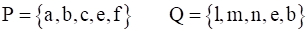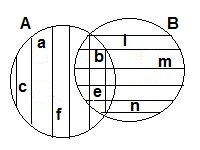Question 17.

Represent the union of two sets by Venn diagram for each of the following.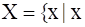is a prime number between 80 and 100}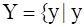is an odd number between 90 and 100}

Given: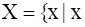is a prime number between 80 and 100}is an odd number between 90 and 100}

X = {83, 89, 93, 97}

Y = {91, 93, 95, 97, 99}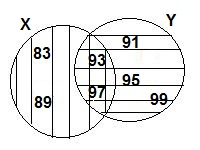Question 18.

Write the subset relations between the following sets.

X = set of all quadrilaterals.

Y = set of all rhombuses.

S = set of all squares.

T = set of all parallelograms.

V = set of all rectangles.

Given: X = set of all quadrilaterals.

Y = set of all rhombuses.

S = set of all squares.

T = set of all parallelograms.

V = set of all rectangles.

S ⊆ X, because all squares are quadrilaterals.

V ⊆ X, because all rectangles are quadrilaterals.

T ⊆ X, because all parallelograms are quadrilaterals.

S ⊆ Y, because all squares are rhombus.

S ⊆ V, because all squares are rectangles.

S ⊆ T, because all squares are parallelograms.

V ⊆ T, because all rectangles are parallelograms.

Y ⊆ T, because all rhombus are parallelograms.

Question 19.

If M is any set, then write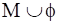and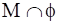Given: M is any set

As we know ϕ is null set.

Hence,

(M ∪ ϕ ) = M

(M ∩ ϕ ) = ϕ

Question 20.

Observe the Venn diagram and write the given sets U,A,B,A ∪ B and A ∩ B.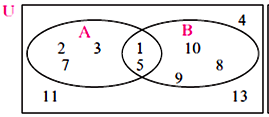(i) U = {1, 2, 3, 4, 5, 7, 8, 9, 10, 11, 13}

(ii) A = {1, 2, 3, 5, 7}

(iii) B = {1, 5, 8, 9, 10}

(iv) (A ∪ B ) = {1, 2, 3, 5, 7, 8, 9, 10}

(v) (A ∩ B ) = {1, 5}

Question 21.

If n(A) = 7, n(B) = 13, n (A ∩ B) =4 then n (A ∪ B) = ?

Given: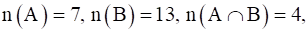As we know, n (A ∪ B) = n(A) + n(B) - n (A ∩ B)

⇒ n (A ∪ B) = 7 + 13 - 4

⇒ n (A ∪ B) = 20 - 4

⇒ n (A ∪ B) = 16

## PDF FILE TO YOUR EMAIL IMMEDIATELY PURCHASE NOTES & PAPER SOLUTION. @ Rs. 50/- each (GST extra)

SUBJECTS

HINDI ENTIRE PAPER SOLUTION

MARATHI PAPER SOLUTION
SSC MATHS I PAPER SOLUTION
SSC MATHS II PAPER SOLUTION
SSC SCIENCE I PAPER SOLUTION
SSC SCIENCE II PAPER SOLUTION
SSC ENGLISH PAPER SOLUTION
SSC & HSC ENGLISH WRITING SKILL
HSC ACCOUNTS NOTES
HSC OCM NOTES
HSC ECONOMICS NOTES
HSC SECRETARIAL PRACTICE NOTES

2019 Board Paper Solution

HSC ENGLISH SET A 2019 21st February, 2019

HSC ENGLISH SET B 2019 21st February, 2019

HSC ENGLISH SET C 2019 21st February, 2019

HSC ENGLISH SET D 2019 21st February, 2019

SECRETARIAL PRACTICE (S.P) 2019 25th February, 2019

HSC XII PHYSICS 2019 25th February, 2019

CHEMISTRY XII HSC SOLUTION 27th, February, 2019

OCM PAPER SOLUTION 2019 27th, February, 2019

HSC MATHS PAPER SOLUTION COMMERCE, 2nd March, 2019

HSC MATHS PAPER SOLUTION SCIENCE 2nd, March, 2019

SSC ENGLISH STD 10 5TH MARCH, 2019.

HSC XII ACCOUNTS 2019 6th March, 2019

HSC XII BIOLOGY 2019 6TH March, 2019

HSC XII ECONOMICS 9Th March 2019

SSC Maths I March 2019 Solution 10th Standard11th, March, 2019

SSC MATHS II MARCH 2019 SOLUTION 10TH STD.13th March, 2019

SSC SCIENCE I MARCH 2019 SOLUTION 10TH STD. 15th March, 2019.

SSC SCIENCE II MARCH 2019 SOLUTION 10TH STD. 18th March, 2019.

SSC SOCIAL SCIENCE I MARCH 2019 SOLUTION20th March, 2019

SSC SOCIAL SCIENCE II MARCH 2019 SOLUTION, 22nd March, 2019

XII CBSE - BOARD - MARCH - 2019 ENGLISH - QP + SOLUTIONS, 2nd March, 2019

HSC Maharashtra Board Papers 2020

(Std 12th English Medium)

HSC ECONOMICS MARCH 2020

HSC OCM MARCH 2020

HSC ACCOUNTS MARCH 2020

HSC S.P. MARCH 2020

HSC ENGLISH MARCH 2020

HSC HINDI MARCH 2020

HSC MARATHI MARCH 2020

HSC MATHS MARCH 2020

SSC Maharashtra Board Papers 2020

(Std 10th English Medium)

English MARCH 2020

HindI MARCH 2020

Hindi (Composite) MARCH 2020

Marathi MARCH 2020

Mathematics (Paper 1) MARCH 2020

Mathematics (Paper 2) MARCH 2020

Sanskrit MARCH 2020

Important-formula

THANKS